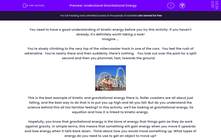# Understand Gravitational Energy

In this worksheet, students will learn about gravitational energy. They will also be introduced to the gravitational energy equation and learn how to apply it to solve problems.Key stage:  KS 4

Year:  GCSE

GCSE Boards:   AQA, AQA Trilogy, AQA Synergy, OCR 21st Century, OCR Gateway, Pearson Edexcel, Eduqas,

Difficulty level:#### Worksheet Overview

You need to have a good understanding of kinetic energy before you try this activity. If you haven't already, it's definitely worth taking a look!

Imagine ...

You're slowly climbing to the very top of the rollercoaster track in one of the cars.  You feel the rush of adrenaline.  You're nearly there and then suddenly, there's nothing.   You look out over the park for a split second and then you plummet, fast, towards the ground.This is the best example of kinetic and gravitational energy there is. Roller coasters are all about just falling, and the best way to do that is to put you up high and let you fall. But do you understand the science behind this all too familiar feeling? In this activity, we'll be looking at gravitational energy, its equation and how it is linked to kinetic energy.

Hopefully, you know that gravitational energy is the store of energy that things gain as they do work against gravity. In simple terms, this means that something will gain energy when you move it upwards and lose energy when it falls back down.  Think about how you would move something up. What types of energy do you need to use to get an object to move up?

If you said kinetic energy, you would be correct! Kinetic energy and gravitational energy have a ‘special relationship’ where kinetic energy needs to be used to give something gravitational energy. The reverse is also true.  If something loses gravitational energy, then it will gain kinetic! Now, because the world isn’t perfect, some of the energy lost from gravitational energy becomes wasted as heat from friction, but it’s a pretty good starting point!

So, what things will affect how much gravitational energy there is? Well, think of the things that will make it harder to lift an object up -  there are three of them (two are obvious and one's a bit trickier).

1  The mass of the object. Okay – so this should make sense, the more massive the object, the harder it is to lift it up. So, that means that energy is proportional to the mass of the object.

Energy (E) ∝ mass (m)

2  The height you lift the object to. You have to use more energy to lift an object higher. So, the higher the object travels, the more energy you need to use to lift that object. Let’s put that into our proportionality.

Energy (E) ∝ mass (m) x height (h)

3  Gravity - this is the tricky one. What if you were to lift that object up on the moon? Would it be easier or harder (assuming you have a spacesuit)? It would be easier because gravity is weaker on the moon than it is on Earth. So, the strength of gravity also affects the gravitational energy. If we put this into our equation, we get something that looks like this:

Gravitational energy = mass x gravitational field strength x height

E = mgh

You will always be told the gravitational field strength in the question.

Now we have the equation, let's go through an example of how to use it:

A roller coaster car has a mass of 15,000 kg and falls a distance of 10 m. How much gravitational energy would it lose during this fall?

Gravitational field strength on Earth = 9.8 N/kg

Step one   Find all of the numbers you need and highlight them:

A roller coaster car has a mass of 15,000 kg and falls a distance of 10 m. How much gravitational energy would it lose during this fall?

Gravitational field strength on Earth = 9.8 N/kg

Step 2  Write out the symbols with their numbers next to them:

m = 15,000 kg

g = 9.8 N/kg

h = 10 m

Step 3  Put the numbers into the equation:

Energy = mass x gravitational field strength x height

E = 15,000 x 9.8 x 10

Step 4  Calculate the equation with a calculator. Write out your answer and don’t forget the unit.

1,470,000 J

All done?  Let’s try out some questions!

### What is EdPlace?

We're your National Curriculum aligned online education content provider helping each child succeed in English, maths and science from year 1 to GCSE. With an EdPlace account you’ll be able to track and measure progress, helping each child achieve their best. We build confidence and attainment by personalising each child’s learning at a level that suits them.

Get started